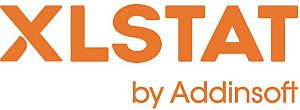# XLSTAT - Cochran-Mantel-Haenszel test

## Use of the Cochran-Mantel-Haenszel test

Use the Cochran-Mantel-Haenszel test to test the hypothesis of independence on a series of contingency tables corresponding to an experiment crossing two categorical variables, with a control variable taking multiple values.

## Principles of the Cochran-Mantel-Haenszel test

Cochran (1954) then Mantel and Haenszel (1959) developed a test that allows to test whether there is independence or not between the rows and columns of the contingency tables, taking into account the fact that the tables are independent of each other (for each dose the patients are different), and by conditioning on the marginal sums of each table, as in the standard test of independence on contingency tables.

The test commonly named the Cochran-Mantel-Haenszel (CMH) test is based on the M² statistic defined by:

M² = [|Σk (n11k- n1+k/ n++k)|-1/2]² / [Σk (n1+k n2+k n+1k n+2k)/( n++k²( n++k²-1))]

This statistic follows asymptotically a chi-square distribution with 1 degree of freedom. Knowing M², we can therefore compute the p-value, and knowing the risk of Type I, alpha, we can determine the critical value. It is also possible, as for the test of independence on a contingency table, to calculate the exact p-value, if the contingency tables are of size 2x2. The use of absolute value and the subtraction of -1 / 2 and the division by (n²++k-1) instead of n²++k corresponds to a continuity correction proposed by Mantel and Haenszel. Its use is strongly recommended. With XLSTAT you have the choice to use it (default) or not.

It may be noted that the numerator measures for the upper left cell the difference between the actual value and the expected value corresponding to independence, and that then sum these differences. If the differences are in opposite directions from one table to another we could therefore conclude that there is independence while there is dependence in each table (Type II error). This situation happens when there is a three-way interaction between the three variables. This test is to be used with caution.

The Cochran-Mantel-Haenszel test has been generalized by Birch (1965), Landis et al. (1978) and Mantel and Byar (1978) to the case of RxC contingency tables where R and C can be greater than 2. The computation of M² is more complex, but it still leads to a statistic that asymptotically follows a chi-square with (L-1) (C-1) degrees of freedom.

It is recommended to perform separately from the CMH test, the analysis of the Cramer's V for the individual contingency tables to get an idea of their contribution to independence. XLSTAT displays automatically for each contingency table, a table with the Cramer's V, the chi-square and the corresponding p-values (exact for 2x2 tables and asymptotic for higher dimensional tables) where possible, that is, when there are no null marginal sums.This analysis is available in the XLStat-Basic addin for Microsoft ExcelKovach Computing Services (KCS) was founded in 1993 by Dr. Warren Kovach. The company specializes in the development and marketing of inexpensive and easy-to-use statistical software for scientists, as well as in data analysis consulting.

### Get in Touch

• Email:
sales@kovcomp.com